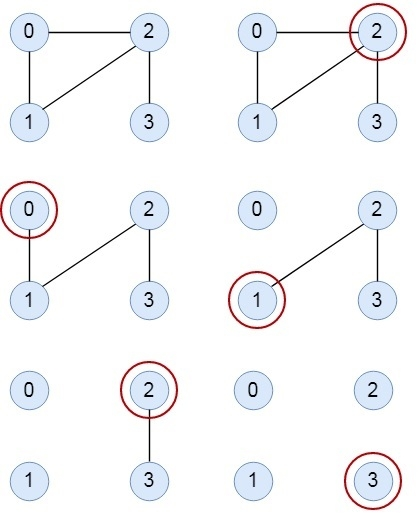# Fleury’s Algorithm for printing Eulerian Path or Circuit in C++

C++Server Side ProgrammingProgramming

Fleury’s Algorithm is used to display the Euler path or Euler circuit from a given graph. In this algorithm, starting from one edge, it tries to move other adjacent vertices by removing the previous vertices. Using this trick, the graph becomes simpler in each step to find the Euler path or circuit.We have to check some rules to get the path or circuit −

• The graph must be a Euler Graph.
• When there are two edges, one is bridge, another one is non-bridge, we have to choose non-bridge at first.s

Choosing of starting vertex is also tricky, we cannot use any vertex as starting vertex, if the graph has no odd degree vertices, we can choose any vertex as start point, otherwise when one vertex has odd degree, we have to choose that one first.

Input − Adjacency matrix of a graph

 0 1 1 1 1 1 0 1 1 1 1 1 0 1 1 1 1 1 0 1 1 1 1 1 0

Output − Euler Path or Circuit: 1--0 0--2 2--1 1--3 3--0 0--4 4--3 3—2

## Algorithm

findStartVert(graph)
Input: The given graph.
Output: Find the starting vertex to start algorithm.
Begin
for all vertex i, in the graph, do
deg := 0
for all vertex j, which are adjacent with i, do
deg := deg + 1
done
if deg is odd, then
return i
done
when all degree is even return 0
End
isBridge(u, v)
Input: The start and end node.
Output: True when u and v are forming a bridge.
Begin
deg := 0
for all vertex i which are adjacent with v, do
deg := deg + 1
done
if deg > 1, then
return false
return true
End
fleuryAlgorithm(start)
Input: The starting vertex.
Output: Display the Euler path or circuit.
Begin
edge := get the number of edges in the graph //it will not initialize in next
recursion call
for all vertex v, which are adjacent with start, do
if edge <= 1 OR isBridge(start, v) is false, then
display path from start and v
remove edge (start,v) from the graph
decrease edge by 1
fleuryAlgorithm(v)
done
End

## Example

Live Demo

#include<iostream>
#include<vector>
#define NODE 5
using namespace std;
int graph[NODE][NODE] = {{0, 1, 1, 1, 1},
{1, 0, 1, 1, 0},
{1, 1, 0, 1, 0},
{1, 1, 1, 0, 1},
{1, 0, 0, 1, 0}
};
int tempGraph[NODE][NODE];
int findStartVert(){
for(int i = 0; i<NODE; i++){
int deg = 0;
for(int j = 0; j<NODE; j++){
if(tempGraph[i][j])
deg++; //increase degree, when connected edge found
}
if(deg % 2 != 0) //when degree of vertices are odd
return i; //i is node with odd degree
}
return 0; //when all vertices have even degree, start from 0
}
bool isBridge(int u, int v){
int deg = 0;
for(int i = 0; i<NODE; i++)
if(tempGraph[v][i])
deg++;
if(deg>1){
return false; //the edge is not forming bridge
}
return true; //edge forming a bridge
}
int edgeCount(){
int count = 0;
for(int i = 0; i<NODE; i++)
for(int j = i; j<NODE; j++)
if(tempGraph[i][j])
count++;
return count; //count nunber of edges in the graph
}
void fleuryAlgorithm(int start){
static int edge = edgeCount();
for(int v = 0; v<NODE; v++){
if(tempGraph[start][v]){ //when (u,v) edge is presnt and not forming bridge
if(edge <= 1 || !isBridge(start, v)){
cout << start << "--" << v << " ";
tempGraph[start][v] = tempGraph[v][start] = 0; //remove edge from graph
edge--; //reduce edge
fleuryAlgorithm(v);
}
}
}
}
int main(){
for(int i = 0; i<NODE; i++) //copy main graph to tempGraph
for(int j = 0; j<NODE; j++)
tempGraph[i][j] = graph[i][j];
cout << "Euler Path Or Circuit: ";
fleuryAlgorithm(findStartVert());
}

## Output

Euler Path Or Circuit: 1--0 0--2 2--1 1--3 3--0 0--4 4--3 3—2